StatLect

# Log-likelihood

The log-likelihood is, as the term suggests, the natural logarithm of the likelihood.## But what is the likelihood?

To define the likelihood we need two things:

1. some observed data (a sample), which we denote by(the Greek letter xi);

2. a set of probability distributions that could have generated the data; each distribution is identified by a parameter(the Greek letter theta).

Roughly speaking, the likelihood is a functionthat gives us the probability of observing the samplewhen the data is extracted from the probability distribution with parameter.

## Example

We will provide below a rigorous definition of log-likelihood, but it is probably a good idea to start with an example.

The typical example is the log-likelihood of a sample of independent and identically distributed draws from a normal distribution.

In this case, the sampleis a vector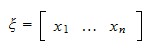whose entriesare draws from a normal distribution.

The probability density function of a drawis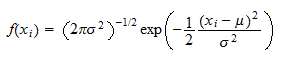whereandare the parameters (mean and variance) of the normal distribution.

The parameter vector isThe set of distributions that could have generated the sample is assumed to be the set of all normal distributions (that can be obtained by varying the parametersand).

In order to stress the fact that the probability density depends on the two parameters, we write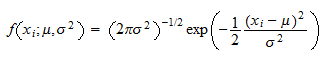The joint probability density of the sampleis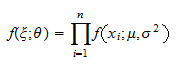because the joint density of a set of independent variables is equal to the product of their marginal densities (see the lecture on Independent random variables).

The likelihood function isIn other words, when we deal with continuous distributions such as the normal distribution, the likelihood function is equal to the joint density of the sample. We will explain below how things change in the case of discrete distributions.

The log-likelihood function is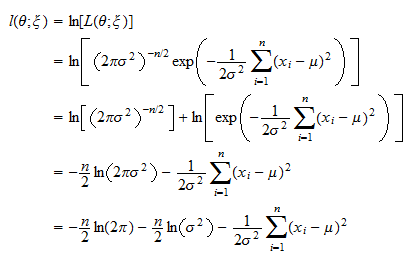## How the log-likelihood is used

The log-likelihood function is typically used to derive the maximum likelihood estimator of the parameter.

The estimatoris obtained by solving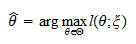that is, by finding the parameterthat maximizes the log-likelihood of the observed sample.

This is the same as maximizing the likelihood functionbecause the natural logarithm is a strictly increasing function.

## Why the log is taken

One may wonder why the log of the likelihood function is taken. There are several good reasons.

To understand them, suppose that the sample is made up of independent observations (as in the example above).

Then, the logarithm transforms a product of densities into a sum. This is very convenient because:

• the asymptotic properties of sums are easier to analyze (one can apply Laws of Large Numbers and Central Limit Theorems to these sums; see the proofs of consistency and asymptotic normality of the maximum likelihood estimator);

• products are not numerically stable: they tend to converge quickly to zero or to infinity, depending on whether the densities of the single observations are on average less than or greater than 1; sums are instead more stable from a numerical standpoint; this is important because the maximum likelihood problem is often solved numerically on computers where limited machine precision does not allow us to distinguish a very small number from zero and a very large number from infinity.

## A rigorous definition

We finally give a rigorous definition of log-likelihood

The following elements are needed to define the log-likelihood function:

• we observe a sample, which is regarded as the realization of a random vector(capital Xi), whose distribution is unknown;

• the distribution ofbelongs to a parametric family: there is a setof real vectors (called the parameter space) whose elements (called parameters) are put into correspondence with the distributions that could have generated; in particular:

• ifis an continuous random vector, its joint probability density function belongs to a set of joint probability density functionsindexed by the parameter;

• ifis a discrete random vector, its joint probability mass function belongs to a set of joint probability mass functionsindexed by the parameter;

• when the joint probability mass (or density) function is considered as a function offor fixed(i.e., for the samplewe have observed), it is called likelihood (or likelihood function) and it is denoted by. So,ifis discrete andifis continuous.

Given all these elements, the log-likelihood function is the functiondefined by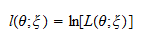## Negative log-likelihood

You will often hear the term "negative log-likelihood". It is just the log-likelihood function with a minus sign in front of it:It is frequently used because computer optimization algorithms are often written as minimization algorithms.

As a consequence, the maximization problemis equivalently written in terms of the negative log-likelihood as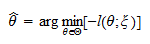before being solved numerically on computers.

## More examples

More examples of how to derive log-likelihood functions can be found in the lectures on:

## More details

The log-likelihood and its properties are discussed in a more detailed manner in the lecture on maximum likelihood estimation.

## Keep reading the glossary

Previous entry: Joint probability mass function

Next entry: Loss function